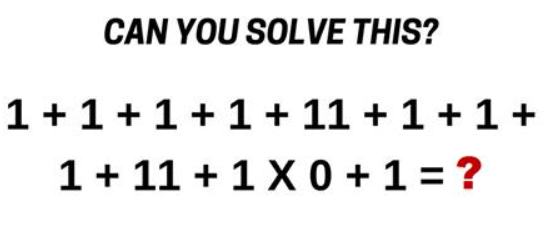﻿ Practice with Order of Operations

#Practice with Order of Operations

The order that operations are to be performed (when not clearly identified) is summarized with the following memory device:

Please Excuse My Dear Aunt Sally
(PEMDAS)

• Do things inside Parentheses first (using PEMDAS, if needed, inside the parentheses).
• Then do all Exponents, in order as they occur, going from left to right.
• Then do all Multiplications/Divisions (they have equal weight) in order as they occur, going from left to right.
• Finally, do all Additions/Subtractions (they have equal weight) in order as they occur, going from left to right.

In September 2017, this problem was floating around the web:Here's the solution, with correct order of operations:

\begin{align} &\cssId{s19}{1 + 1 + 1 + 1 + 11 + 1 + 1 + 1 + 11 + 1\times 0 + 1} \cr\cr &\quad\cssId{s20}{= 1 + 1 + 1 + 1 + 11 + 1 + 1 + 1 + 11 + (1\times 0) + 1}\cr &\quad\quad\cssId{s21}{\text{(the multiplication gets done first)}} \cr\cr &\quad\cssId{s22}{= 1 + 1 + 1 + 1 + 11 + 1 + 1 + 1 + 11 + 0 + 1}\cr\cr &\quad\cssId{s23}{= 30} \end{align}

If this doesn't make sense to you, try the following mental exercise on a shorter (but similar) problem: $$\cssId{s25}{1 + 2 \times 0 + 3}$$

• Replace each plus sign with an (equally-weak) person.
• Replace the multiplication sign with a strong person. Why? Multiplication is ‘stronger than’ addition! And this makes perfectly good sense, since multiplication is ‘super-addition’. For example: $$\cssId{s31}{\,5\times 2 = 2 + 2 + 2 + 2 + 2}$$
• The first (left-most) weak guy is trying to pull together the $\,1\,$ and the $\,2\,,$ to add them.
• The middle (strong) guy is trying to pull together the $\,2\,$ and the $\,0\,,$ to multiply them.
• The right-most weak guy is trying to pull together the $\,0\,$ and the $\,3\,,$ to add them.
• Who wins? Clearly, the strong guy!

1203

\begin{align} &\cssId{s37}{1 + 2 \times 0 + 3}\cr &\qquad\cssId{s38}{=\ \ 1 + \overbrace{(2\times 0)}^{\text{strong guy wins}} + 3}\cr\cr &\qquad\cssId{s39}{=\ \ 1 + 0 + 3}\cr\cr &\qquad\cssId{s40}{=\ \ 4} \end{align}

## More Examples

\begin{align} &\cssId{s42}{-1 + 3\times 5 - 2}\cr\cr &\qquad\cssId{s43}{= -1 + (3\times 5) - 2}\cr\cr &\qquad\cssId{s44}{= 12} \end{align}
\begin{align} &\cssId{s45}{2 - 10\div 5 + 3}\cr\cr &\qquad\cssId{s46}{= 2 - \frac{10}{5} + 3}\cr\cr &\qquad\cssId{s47}{= 3} \end{align}

Curious readers may want to explore the optional section: Laurel, Yanny, Cookies, Bananas, and Clocks

## Practice

Feel free to use a pencil and scrap paper to work these problems. However, do not use your calculator!